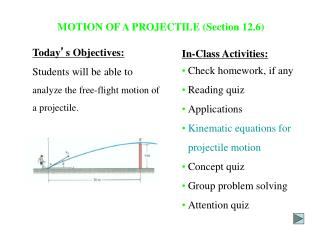Download PresentationMOTION OF A PROJECTILE (Section 12.6)

# MOTION OF A PROJECTILE (Section 12.6) - PowerPoint PPT PresentationDownload Presentation## MOTION OF A PROJECTILE (Section 12.6)

- - - - - - - - - - - - - - - - - - - - - - - - - - - E N D - - - - - - - - - - - - - - - - - - - - - - - - - - -
##### Presentation Transcript

1. MOTION OF A PROJECTILE (Section 12.6) Today’s Objectives: Students will be able to analyze the free-flight motion of a projectile. In-Class Activities: • Check homework, if any • Reading quiz • Applications •Kinematic equations for projectile motion • Concept quiz • Group problem solving • Attention quiz

2. READING QUIZ • The downward acceleration of an object in free-flight motion is • A) zero B) increasing with time • C) 9.81 m/s2 D) 9.81 ft/s2 2. The horizontal component of velocity remains _________ during a free-flight motion. A) zero B) constant C) at 9.81 m/s2 D) at 32.2 ft/s2

3. APPLICATIONS A kicker should know at what angle, q, and initial velocity, vo, he must kick the ball to make a field goal. For a given kick “strength”, at what angle should the ball be kicked to get the maximum distance?

4. APPLICATIONS (continued) A fireman wishes to know the maximum height on the wall he can project water from the hose. At what angle, q, should he hold the hose?

5. For illustration, consider the two balls on the left. The red ball falls from rest, whereas the yellow ball is given a horizontal velocity. Each picture in this sequence is taken after the same time interval. Notice both balls are subjected to the same downward acceleration since they remain at the same elevation at any instant. Also, note that the horizontal distance between successive photos of the yellow ball is constant since the velocity in the horizontal direction is constant. CONCEPT OF PROJECTILE MOTION Projectile motion can be treated as two rectilinear motions, one in the horizontal direction experiencing zero acceleration and the other in the vertical direction experiencing constant acceleration (i.e., gravity).

6. KINEMATIC EQUATIONS: HORIZONTAL MOTION Since ax = 0, the velocity in the horizontal direction remains constant (vx = vox) and the position in the x direction can be determined by: x = xo + (vox)(t) Why is ax equal to zero (assuming movement through the air)?

7. KINEMATIC EQUATIONS: VERTICAL MOTION Since the positive y-axis is directed upward, ay = -g. Application of the constant acceleration equations yields: vy = voy – g(t) y = yo + (voy)(t) – ½g(t)2 vy2 = voy2 – 2g(y – yo) For any given problem, only two of these three equations can be used. Why?

8. Solution:Using vx = vo cos θ and vy = vo sin θ We can write: x = (vo cos θ)t or y = (vo sin θ)t – ½ g(t)2 x t = vo cos θ By substituting for t: 2 ( ) ( ) ( ) x g x – y = (vo sin θ) vo cos θ 2 vo cos θ Example 1 Given: vo and θ Find:The equation that defines y as a function of x. Plan: Eliminate time from the kinematic equations.

9. Simplifying the last equation, we get: ( ) gx2 y = (x tanq) – (1 + tan2q) 2vo2 Example 1 (continued): The above equation is called the “path equation” which describes the path of a particle in projectile motion. The equation shows that the path is parabolic.

10. Given: Snowmobile is going 15 m/s at point A. Find: The horizontal distance it travels (R) and the time in the air. Solution: First, place the coordinate system at point A. Then write the equation for horizontal motion. + xB = xA + vAxtAB and vAx = 15 cos 40° m/s Now write a vertical motion equation. Use the distance equation. + yB = yA + vAytAB – 0.5gctAB2 vAy = 15 sin 40° m/s Note that xB = R, xA = 0, yB = -(3/4)R, and yA = 0. Example 2 Solving the two equations together (two unknowns) yields R = 19.0 m tAB = 2.48 s

11. CONCEPT QUIZ 1. In a projectile motion problem, what is the maximum number of unknowns that can be solved? A) 1 B) 2 C) 3 D) 4 2. The time of flight of a projectile, fired over level ground with initial velocity Vo at angle θ, is equal to A) (vo sin q)/g B) (2vo sin q)/g C) (vo cos q)/g D) (2vo cos q)/g

12. Given: Skier leaves the ramp at qA = 25o and hits the slope at B. Find: The skier’s initial speed vA. GROUP PROBLEM SOLVING Plan: Establish a fixed x,y coordinate system (in the solution here, the origin of the coordinate system is placed at A). Apply the kinematic relations in x and y-directions.

13. Motion in x-direction: Using xB = xA + vox(tAB) (4/5)100 88.27 tAB= = vA (cos 25) vA Motion in y-direction: Using yB = yA + voy(tAB) – ½ g(tAB)2 2 80 88.27 -64 = 0 + vA(sin 45) – ½ (9.81) vA vA (cos 25) vA = 19.42 m/s GROUP PROBLEM SOLVING (continued) Solution:

14. ATTENTION QUIZ 1. A projectile is given an initial velocity vo at an angle f above the horizontal. The velocity of the projectile when it hits the slope is ____________ the initial velocity vo. A) less than B) equal to C) greater than D) None of the above. 2. A particle has an initial velocity vo at angle q with respect to the horizontal. The maximum height it can reach is when A) q = 30° B) q = 45° C) q = 60° D) q = 90°

15. End of the Lecture Let Learning Continue